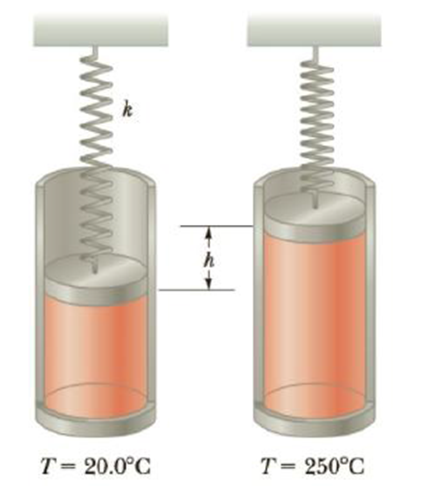Chapter 10, Problem 64AP

Chapter
Section
Textbook Problem

An expandable cylinder has its top connected to a spring with force constant 2.00 × 103 N/m (Fig. P10.64). The cylinder is Filled with 5.00 L of gas with the spring relaxed at a pressure of 1.00 atm and a temperature of 20.0°C. (a) If the lid has a cross-sectional area of 0.010 0 m2 and negligible mass, how high will the lid rise when the temperature is raised to 250°C? (b) What is the pressure of the gas at 250°C?Figure P10.64

(a)

To determine
The height up to which the lid rises.

Explanation

Given info:

The initial pressure is 1.0 atm.

The initial volume is 5.0 L.

The initial temperature is 20οC .

The final temperature is 250οC .

The force constant is 2×103N/m .

The cross sectional area is 0.010m2 .

Explanation:

From Ideal gas law,

(P2P1)(V2V1)=T2T1 (I)

• P1 and P2 are the initial and final pressures.
• T1 and T2 are the initial and final temperatures.
• V1 and V2 are the initial and final volumes.

Formula to calculate the final pressure is,

P2=P1+(khA) (II)

• k is the force constant.
• h is the height up to which the lid rises.
• A is the cross sectional area.

Formula to calculate the final volume is,

V2=V1+Ah (III)

Substitute Equations (II) and (III) in (I)

(1+khAP1)(1+AhV1)=T2T1

On Re-arranging the above equation,

(kP1V1)h2+(kAP1+AV1)h+(1T2T1)=0

Using the quadratic formula to solve for h,

h=(kAP1+AV1)±(kAP1+AV1)24(kP1V1)(1T2T1)2(kP1V1)

Substitute 1

(b)

To determine
The pressure of the gas at 250οC .

Still sussing out bartleby?

Check out a sample textbook solution.

See a sample solution

The Solution to Your Study Problems

Bartleby provides explanations to thousands of textbook problems written by our experts, many with advanced degrees!

Get Started

Find more solutions based on key concepts